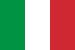# Where Does The Money Go Testo

Traduzione di Where Does The Money Go## Testo Where Does The Money Go

Where does the money go, where does the money go?
Where does the money go, where does the money go?

Always someone else to pay
Bills get more and more each day
What am I supposed to do?

Good to hold but hard to keep
Modern life it don't come cheap
Don't know how I'm gonna get through
You win some, you lose some
It makes you, it breaks you

Where does the money go, where does the money go?
Everyday you look you've spent it
Where does the money go, where does the money go?
Time and time again
Where does the money go, where does the money go?
The problem is never ending
Once you got it in your pocket
Need to find a way to lock it

We all buy more than we need
And the paying off is hard

Pink champagne, the finer things
Who cares what tomorrow brings?
Put it on the credit card
You win some, you lose some
It makes you, it breaks you

Where does the money go, where does the money go?
Everyday you look you've spent it
Where does the money go, where does the money go?
Time and time again
Where does the money go, where does the money go?
The problem is never ending
Once you got it in your pocket
Need to find a way to lock it

Would we be better off without it?
Do we really need it?
Some people give it all away

Where does the money go, where does the money go?
Everyday you look you've spent it
Where does the money go, where does the money go?
Time and time again
Where does the money go, where does the money go?
The problem is never ending
Once you got it in your pocket
Need to find a way to lock it

Where does the money go, where does the money go?
Everyday you look you've spent it
Where does the money go, where does the money go?
Time and time
Time and time again
Where does the money go, where does the money go?
The problem is never ending
Once you got it in your pocket
Need to find a way to lock it away

Where does the money go, where does the money go?
Everyday you look you've spent it
Where does the money go, where does the money go?
Ti-ti-time and time again
Where does the money go, where does the money go?
The problem is never ending
Once you got it in your pocket
Need to find a way to lock it away

Where does the money go, where does the money go?
(I wanna know, I wanna know...)
Where does the money go, where does the money go?
(Where does the money go, where does the money go?)